# Python OpenCV 图像处理再循环，第一阶段复盘Python OpenCV 365 天学习计划，与橡皮擦一起进入图像领域吧。本篇博客是这个系列的第 40 篇。

## 图像中的像素

import cv2 as cvimage = cv.imread("./test.jpg")cv.imshow("image",image)# 输出像素print(image.shape)print(image[:5,:])cv.waitKey(0)cv.destroyAllWindows()

(640, 640, 3)[[[212 151  87]  [175 104  41]  [231 137  78]  ...  [150 144 145]  [188 186 132]  [196 195 115]] [[255 229 160]  [188 118  51]  [196 106  42]  ...  [206 190 178]  [216 200 153]  [199 183 117]]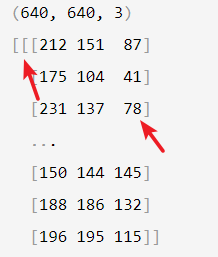### 获取单通道数据与像素值的加减操作

print(image[:5,:,0])

[[212 175 231 ... 150 188 196] [255 188 196 ... 206 216 199] [251 180 201 ... 233 236 220] [173 182 232 ... 237 244 236] [187 210 233 ... 255 255 235]]

import cv2 as cvfrom matplotlib import pyplot as pltimage = cv.imread("./test.jpg")cv.imshow("image", image)# 输出像素print(image.shape)image[:, :, 0] = 0image[:, :, 1] = 0r = image[:, :, 2]cv.imshow("dst",image)cv.imshow("r",r)cv.waitKey(0)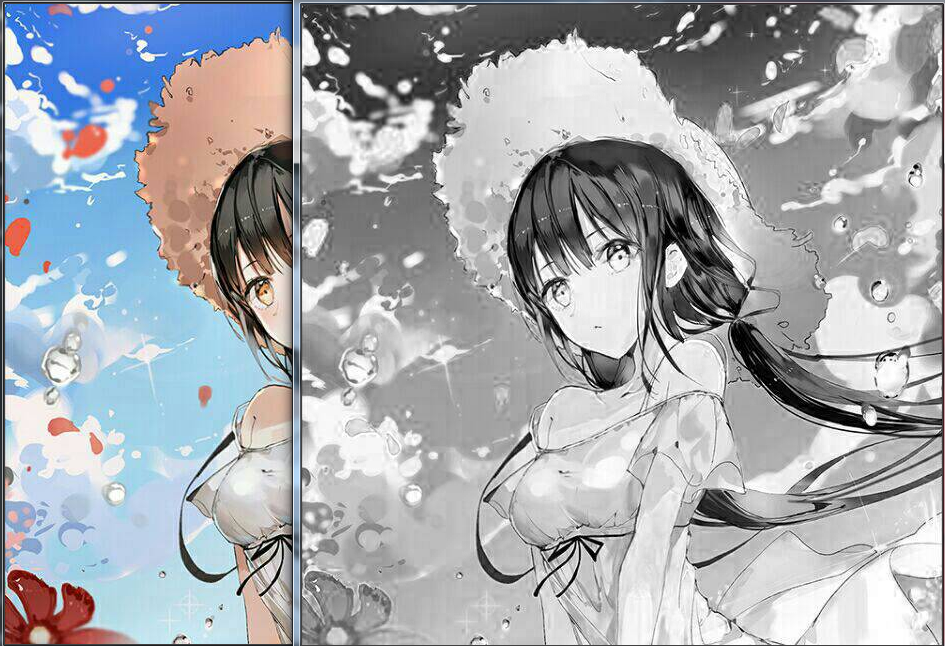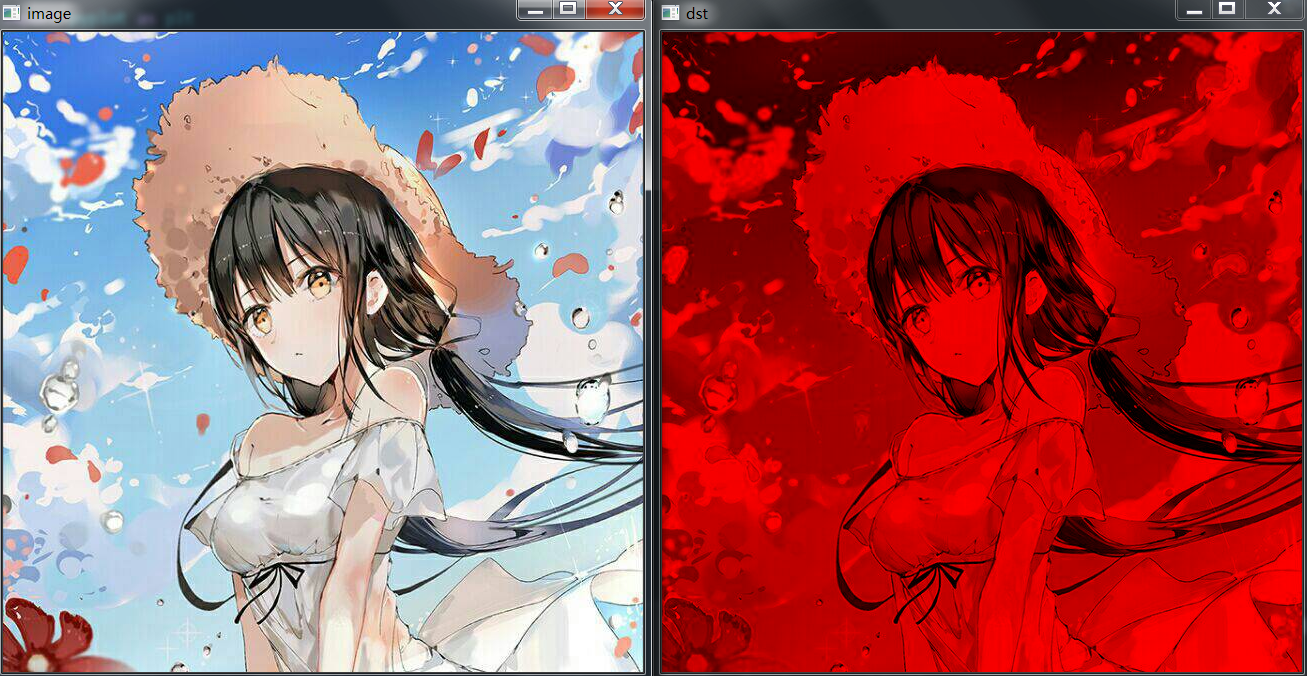image[:, :, 0] = 0cv.imshow("dst",image)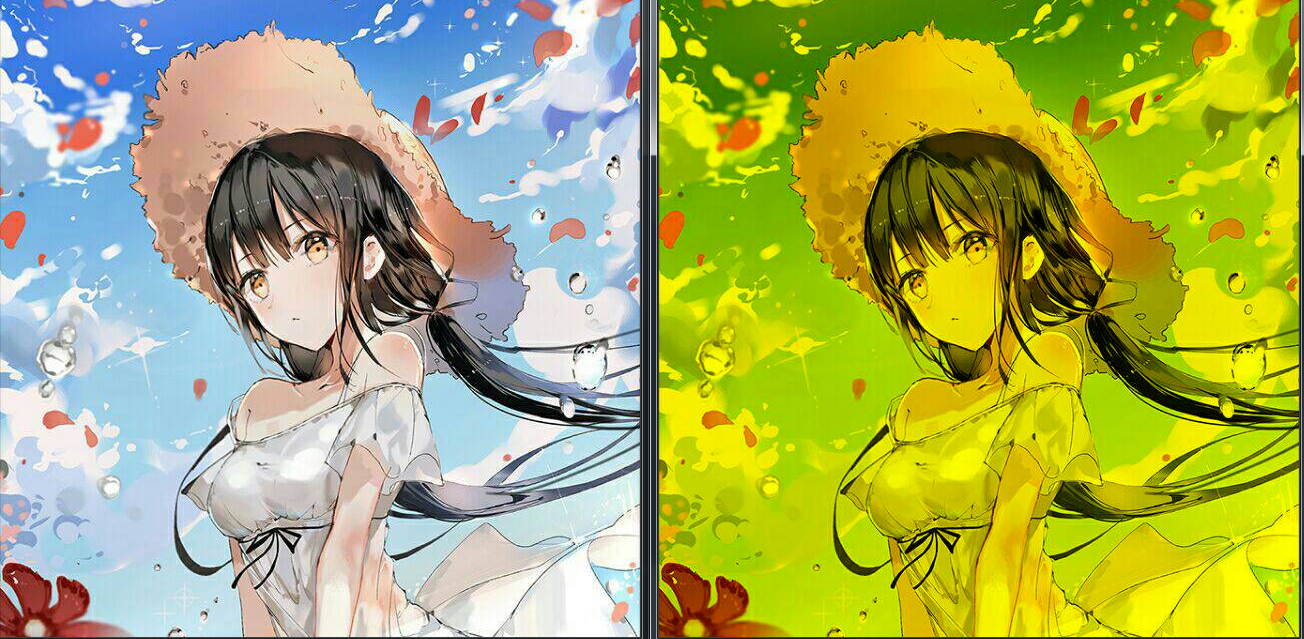import cv2 as cvfrom matplotlib import pyplot as pltimage = cv.imread("./test.jpg")cv.imshow("image", image)print(image[:1, :])print("像素值+10")image = image+10print(image[:1, :])cv.imshow("dst", image)cv.waitKey(0)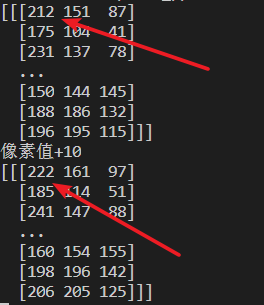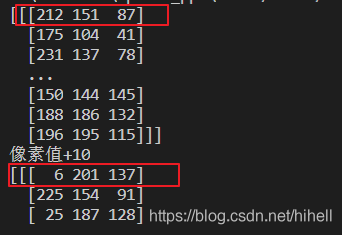### 边界填充

dst = cv2.copyMakeBorder(src, top, bottom, left, right, borderType[, dst[, value]])

borderType 具体取值如下：

• cv2.BORDER_CONSTANT：添加的边界框像素值为常数（需要额外再给定一个参数，就是 value 参数）；

• cv2.BORDER_REFLECT：添加的边框像素将是边界元素的镜面反射，类似于 gfedcb|abcdefgh|gfedcba，注意竖线左右的值即可；

• cv2.BORDER_REFLECT_101 or cv2.BORDER_DEFAULT：和上面类似，但是有一些细微的不同，类似于 gfedcb|abcdefgh|gfedcba，注意竖线左右的数值，没有 a

• cv2.BORDER_REPLICATE：使用最边界的像素值代替，类似于 aaaaaa|abcdefgh|hhhhhhh，直接延伸边界像素值；

• cv2.BORDER_WRAPcdefgh|abcdefgh|abcdefg。具体的你可以在 官方手册 继续学习。最后一个 value 参数：如果 borderTypecv2.BORDER_CONSTANT 时需要填充的常数值。

dst：添加完边框的新图像，需要记住图像的新尺寸为  (src.shape+left+right, src.shape+top+bottom)。通过下述代码，进行完成的呈现。

import cv2 as cvfrom matplotlib import pyplot as pltimage = cv.imread("./t1.png")b, g, r = cv.split(image)rgb_img = cv.merge((r, g, b))replicate = cv.copyMakeBorder(rgb_img, 100, 100, 100, 100, cv.BORDER_REPLICATE)reflect = cv.copyMakeBorder(rgb_img, 100, 100, 100, 100, cv.BORDER_REFLECT)reflect101 = cv.copyMakeBorder(    rgb_img, 100, 100, 100, 100, cv.BORDER_REFLECT_101)wrap = cv.copyMakeBorder(rgb_img, 100, 100, 100, 100, cv.BORDER_WRAP)constant = cv.copyMakeBorder(    rgb_img, 100, 100, 100, 100, cv.BORDER_CONSTANT, value=(0, 255, 0))plt.subplot(231), plt.imshow(rgb_img), plt.title('ORIGINAL')plt.subplot(232), plt.imshow(replicate), plt.title('REPLICATE')plt.subplot(233), plt.imshow(reflect), plt.title('REFLECT')plt.subplot(234), plt.imshow(reflect101), plt.title('REFLECT_101')plt.subplot(235), plt.imshow(wrap), plt.title('WRAP')plt.subplot(236), plt.imshow(constant), plt.title('CONSTANT')plt.show()cv.waitKey(0)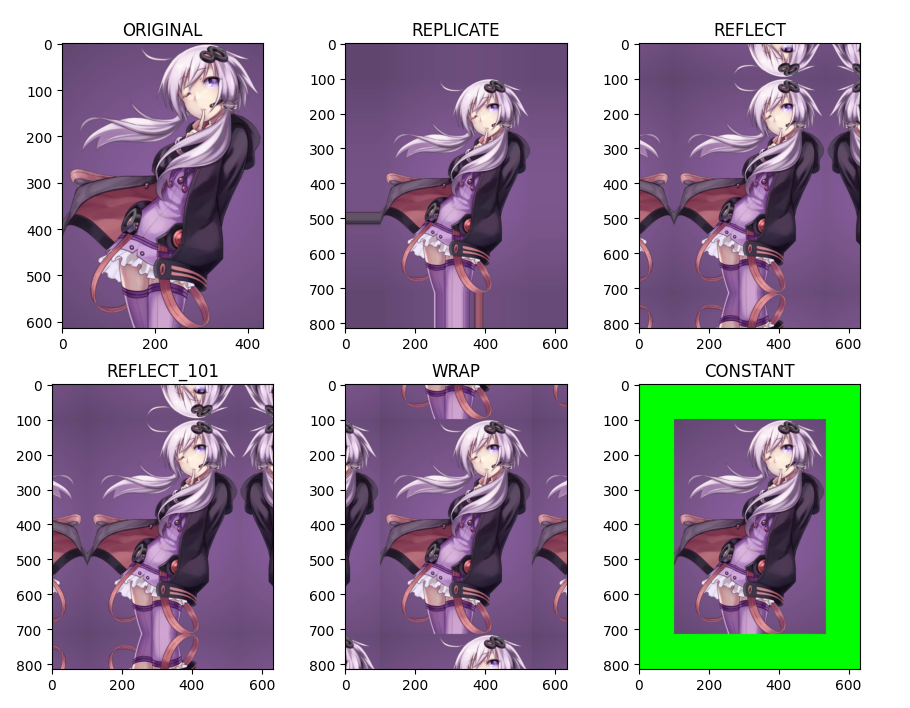## 橡皮擦的小节### 梦想橡皮擦

6 年产品经理+教学经验，3 年互联网项目管理经验； 互联网资深爱好者； 沉迷各种技术无法自拔，导致年龄被困在 25 岁； CSDN 爬虫 100 例作者。 个人公众号“梦想橡皮擦”。

## 评论